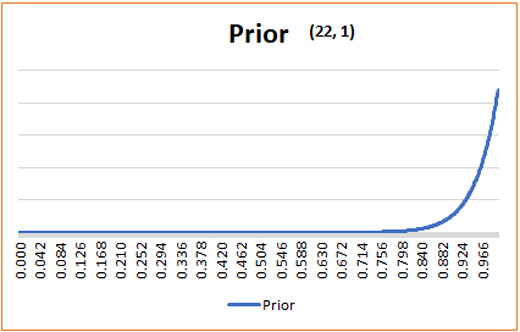#Quality Digest

Featured Product
This Week in Quality Digest Live
Lean Features
Sarah Burlingame
Coaching can keep management and employees on track
Michaël Bikard
Receiving outsized credit can encourage individuals to work together, even when it results in lower-quality output.
Rachel Gordon
New algorithm is accurate, efficient, and adaptable for complex, real-world assemblies
Sue Via
Brian Brooks
We talk prevention, but do detection
Lean News
Quality doesn’t have to sacrifice efficiency
Weighing supply and customer satisfaction
Specifically designed for defense and aerospace CNC machining and manufacturing
From excess inventory and nonvalue work to \$2 million in cost savings
Tactics aim to improve job quality and retain a high-performing workforce
Sept. 28–29, 2022, at the MassMutual Center in Springfield, MA
Enables system-level modeling with 2D and 3D visualization, reducing engineering effort, risk, and cost
It is a smart way to eliminate waste and maximize value
Simplified process focuses on the fundamentals every new ERP user needsBio

Lean

## Reliability Sample Size Calculation Based on Bayesian Inference

### When is enough, enough? That depends.

Published: Monday, July 2, 2018 - 11:03

I have written about sample size calculations many times before. One of the most common questions a statistician is asked is, “How many samples do I need—is a sample size of 30 appropriate?” The appropriate answer to such a question is always, “It depends!”

In today’s column, I have attached a spreadsheet that calculates the reliability based on Bayesian Inference. Ideally, one would want to have some confidence that the widgets being produced is X-percent reliable, or in other words, it is X-percent probable that the widget would function as intended. The ubiquitous 90/90 or 95/95 confidence/reliability sample size table is used for this purpose.In Bayesian Inference, we do not assume that the parameter (i.e., the value that we are calculating, like reliability) is fixed. In the non-Bayesian (or frequentist) world, the parameter is assumed to be fixed, and we need to take many samples of data to make an inference regarding the parameter. For example, we may flip a coin 100 times and calculate the number of heads to determine the probability of heads with the coin (if we believe it is a loaded coin).

In the non-Bayesian world, we may calculate confidence intervals. However, the confidence interval does not provide a lot of practical value. My favorite explanation for confidence interval is with the analogy of an archer. Let’s say that the archer shot an arrow, and it hit the bull’s-eye. We can draw a 3-in. circle around this and call that our confidence interval, based on the first shot. Now let’s assume that the archer shot 99 more arrows, and they all missed the bull’s-eye. For each shot, we drew a 3-in. circle around the hit, resulting in 100 circles. A 95-percent confidence interval simply means that 95 of the circles drawn contain the first bull’s-eye that we drew. In other words, if we repeated the study a lot of times, 95 percent of the confidence intervals calculated will contain the true parameter that we are after. This would indicate that the one study we did may or may not contain the true parameter.

Compared to this, in the Bayesian world, we calculate the credible interval. In a practical sense, this means that we can be 95-percent confident that the parameter is inside the 95-percent credible interval we calculated.

In the Bayesian world, we can have a prior belief and make an inference based on that belief. However, if your prior belief is very conservative, the Bayesian inference might make a slightly liberal inference. Similarly, if your prior belief is very liberal, the inference made will be slightly conservative. As the sample size goes up, the effect of this prior belief is minimized. A common method in Bayesian inference is to use the uninformed prior. This means that we are assuming equal likelihood for all the events. For a binomial distribution, we can use beta distribution to model our prior belief. We will use (1, 1) to assume the uninformed prior. This is shown below:For example, if we use 59 widgets as our samples, and all of them met the inspection criteria, then we can calculate the 95-percent, lower-bound credible interval as 95.13 percent. This is assuming the (1, 1) beta values.

Now let’s say that we are very confident of the process because we have historical data. Now we can assume a stronger prior belief with the beta values as (22,1). The new prior plot is shown below:Based on this, if we had 0 rejects for the 59 samples, then the 95-percent, lower-bound credible interval is 96.37 percent. A slightly higher reliability is estimated based on the strong prior.

We can also calculate a very conservative case of (1, 22), where we assume very low reliability to begin with. This is shown below:Now when we have 0 rejects with 59 samples, we are pleasantly surprised because we were expecting our reliability to be around 8–10 percent. The newly calculated 95-percent, lower-bound credible interval is 64.9 percent.

I have created a spreadsheet that you can play around with. Enter the data in the yellow cells. For a stronger prior (liberal), enter a higher a_prior value. Similarly, for a conservative prior, enter a higher b_prior value. If you are unsure, retain the (1, 1) value to have a uniform prior. The spreadsheet also calculates the maximum expected rejects per million value as well.

I will finish with my favorite confidence interval joke:

“Excuse me, professor,” asked the student. “Why do we always calculate a 95-percent confidence interval and not a 94-percent or 96-percent interval?”

“Shut up,”  explained the professor.

Always keep on learning....

First published April 22, 2018, on Harish's Notebook.

### Discuss